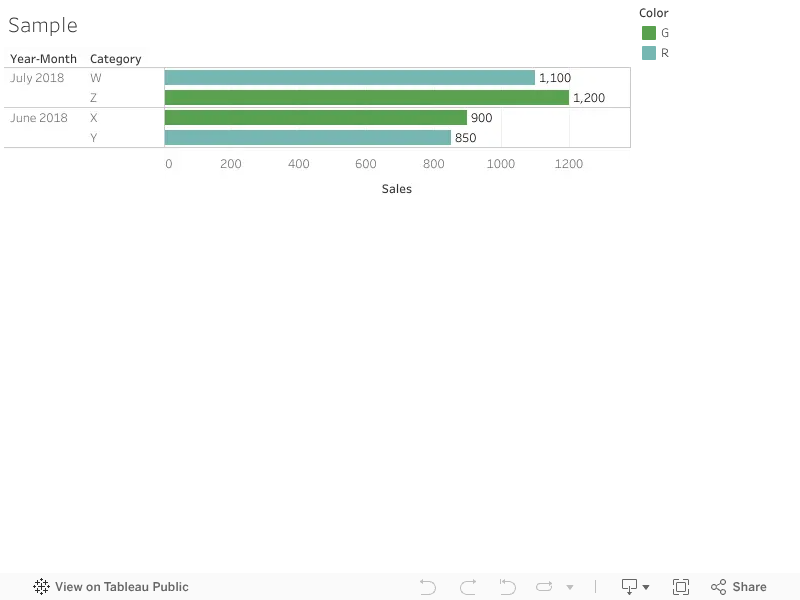6 Replies Latest reply on Jul 26, 2018 8:36 AM by Sandeep Punjari

# Highlight the MAX value in a table with hierarchy

Hi! in my actual project i have the same issue, i have a hierarchy with two levels Category --> Errors. I have a table where i put Year/Month, Category, Errors in the rows wich can be expanded or not expanded in any time and in the columns i put SUM([Sales]).

The client its actually ask my to highlight the MAX value of each posibility, for example:

if i have the table with category being the top level of the hierarchy i have to highlight de max valuesIn the other hand, if i have the next tablei have to hightlight the max values but with the hierarchy expanded,

Any idea on how to do this? i tried with a LOD but cannot succed.

Thanks

Robertino

• ###### 1. Re: Highlight the MAX value in a table with hierarchy

Hello,

Could you please attach a sample workbook.If this is what you are trying to do then:

>Create a calculated field for Max value:

{ FIXED DATETRUNC('month', [Year-Month]):MAX([Sales])}

>Create a calculation for color

IF SUM([Sales])=SUM([max])

THEN

'R'

ELSE

'G'

END

>Drag color field to colors.

add a calculation with numeric 1 add that field to size.

It might solve the issue.

Let me know if it works.

Thanks,

Sandeep

• ###### 2. Re: Highlight the MAX value in a table with hierarchy

Hi Sandeep, thanks for the quick response, im gonna attached the twbx with an example of what i want.

Here is an image, this is the first posibilityUsing a dim like year/month (in this case is not a date dimension, but for the examples is alright), if you see, the hierarchy is not expanded so the year/month and category are in the rows and ordered on descending way. i need to highlight with one color the max value and with other color the other values (in the example in July has to highlight Z category an in June X)

Now, if i expande the hierarchy, tableau shows me for each category the products in it and i need to colour the max value for each combination of category/product with one color and the rest of values with the other color.Z3, W2 in July and X1,Y2 in June

I hope made myself understand.

Robertino

• ###### 3. Re: Highlight the MAX value in a table with hierarchy

Hi Robertino,I think this might help you. Sorry I am using tableau public and don't really know how to attach the workbook.

or you can download it using following link:

This is what I did:

>There are two max calculations:

Max category calculation:

{ FIXED [Year-Month]:MAX({ FIXED [Year-Month],[Category]:SUM([Sales])})}

Max sub category calculation:

{ FIXED [Year-Month],[Category]:MAX({ FIXED [Year-Month],[Category],[Sub-Category]:SUM([Sales])})}

>Color Calculation this is what we need to use in the color:

IF SUM([Sales])=SUM([Max Category]) OR SUM([Sales])=SUM([Max Sub Category])

THEN

'G'

ELSE

'R'

END

Let me know if it works for you.

1 of 1 people found this helpful
• ###### 4. Re: Highlight the MAX value in a table with hierarchy

Perfect Sandeep, the solution goes perfect!

Thank you very much!

Robertino

• ###### 5. Re: Highlight the MAX value in a table with hierarchy

Hi Sandeep, i have another question, because in the real case i have a lot of filters in the data, and the hightlight of the max values isn't working fine,

i attached the workbook in the main topic so you can analyze it, thanks!

• ###### 6. Re: Highlight the MAX value in a table with hierarchy

Hello Robertion,

The Fixed calculation will not consider the values that are in quick filter, for this if the data is not huge we can do one thing is make all the filters to context filter. So that the context filters would execute first and Fixed calculation will include all the filters in it.

Or we might need to add all the fields that are in in filter to the fixed calculation.

For more information:

Order of execution : Tableau's Order of Operations

LOD Expressions:

Let me know if it helps you.

Thanks,

Sandeep